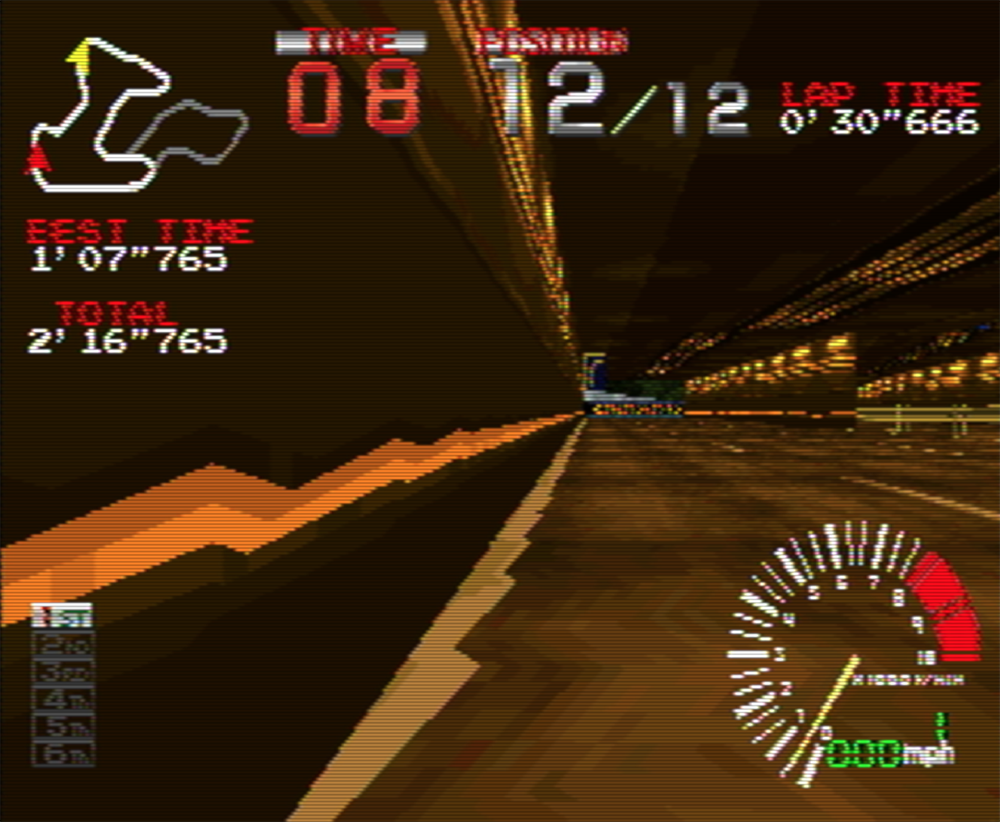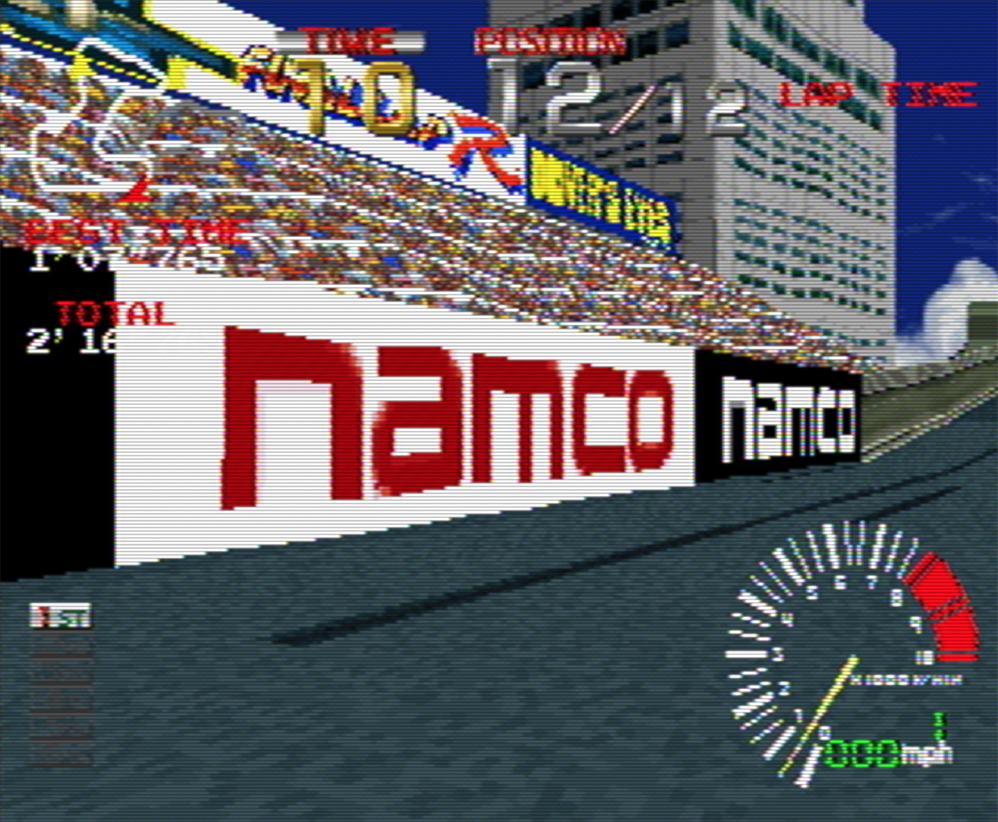# WebGLFundamentals.org

Fix, Fork, Contribute

# WebGL 纹理映射的透视纠正

v_color is interpolated between v0, v1 and v2

``````  // 属性从缓冲中获取数据
attribute vec4 a_position;

// 所有的着色器都有一个 main 函数
void main() {

// gl_Position 是着色器需要设置的一个特殊的变量
gl_Position = a_position;
}
``````

``````  // 片段着色器没有默认的精度，
// 中等精度是个不错的默认值
precision mediump float;

void main() {
// gl_FragColor 是片段着色器需要设置的一个特殊变量
gl_FragColor = vec4(1, 0, 0.5, 1); // 返回红紫色
}
``````

``````var positions = [
-.8, -.8, 0, 1,  // 第一个矩形的第一个三角形
.8, -.8, 0, 1,
-.8, -.2, 0, 1,
-.8, -.2, 0, 1,  // 第一个矩形的第二个三角形
.8, -.8, 0, 1,
.8, -.2, 0, 1,

-.8,  .2, 0, 1,  // 第二个矩形的第一个三角形
.8,  .2, 0, 1,
-.8,  .8, 0, 1,
-.8,  .8, 0, 1,  // 第二个矩形的第二个三角形
.8,  .2, 0, 1,
.8,  .8, 0, 1,
];
``````

``````  attribute vec4 a_position;
+  attribute float a_brightness;

+  varying float v_brightness;

void main() {
gl_Position = a_position;

+    // 直接传递亮度到片段着色器
+    v_brightness = a_brightness;
}
``````

``````  precision mediump float;

+  // 顶点着色器的值插值后传入这里
+  varying float v_brightness;

void main() {
*    gl_FragColor = vec4(v_brightness, 0, 0, 1);  // 红色
}
``````

``````  // 创建缓冲并放入 12 个亮度值
var brightnessBuffer = gl.createBuffer();

// 绑定到 ARRAY_BUFFER
gl.bindBuffer(gl.ARRAY_BUFFER, brightnessBuffer);

var brightness = [
0,  // 第一个矩形的第一个三角形
1,
0,
0,  // 第一个矩形的第二个三角形
1,
1,

0,  // 第二个矩形的第一个三角形
1,
0,
0,  // 第二个矩形的第二个三角形
1,
1,
];

gl.bufferData(gl.ARRAY_BUFFER, new Float32Array(brightness), gl.STATIC_DRAW);
``````

``````  // 找到顶点数据的输入位置
var positionAttributeLocation = gl.getAttribLocation(program, "a_position");
+  var brightnessAttributeLocation = gl.getAttribLocation(program, "a_brightness");
``````

``````  // 启用属性
gl.enableVertexAttribArray(brightnessAttributeLocation);

// 绑定位置缓冲
gl.bindBuffer(gl.ARRAY_BUFFER, brightnessBuffer);

// 告诉属性如何从缓冲中读取数据
var size = 1;          // 每次迭代读取一个单位数据
var type = gl.FLOAT;   // 数据类型是 32 位浮点型
var normalize = false; // 不用单位化
var stride = 0;        // 每次迭代需要移动的距离
var offset = 0;        // 从缓冲的起始处开始
gl.vertexAttribPointer(
brightnessAttributeLocation, size, type, normalize, stride, offset);
``````

``````  var mult = 20;
var positions = [
-.8,  .8, 0, 1,  // 第一个矩形的第一个三角形
.8,  .8, 0, 1,
-.8,  .2, 0, 1,
-.8,  .2, 0, 1,  // 第一个矩形的第二个三角形
.8,  .8, 0, 1,
.8,  .2, 0, 1,

-.8       , -.2       , 0,    1,  // 第二个矩形的第一个三角形
.8 * mult, -.2 * mult, 0, mult,
-.8       , -.8       , 0,    1,
-.8       , -.8       , 0,    1,  // 第二个矩形的第二个三角形
.8 * mult, -.2 * mult, 0, mult,
.8 * mult, -.8 * mult, 0, mult,
];
``````

``````gl_FragColor = vec4(fract(v_brightness * 10.), 0, 0, 1);  // 红色
``````

`v_brightness` 乘以 10 就会是值的范围为 0 到 10，`fract` 会保留小数部分所以结果还是 0 到 1之间， 但是总共是 10 次 0 到 1 了。

`````` result = (1 - t) * a + t * b
``````

`t` 的范围是 0 到 1，表示 `a``b` 之间的位置，0 表示在 `a` 点，1 表示在 `b` 点。

`````` result = (1 - t) * a / aW + t * b / bW
-----------------------------
(1 - t) / aW + t / bW
``````

`aW` 表示 `a` 点的 `W` 值，也就是 `gl_Position.w` 的值，而不一定等于缓冲中的值， 同理 `bW` 是设置在 `b` 点的 `gl_Position.w`

``````attribute vec4 a_position;
attribute vec2 a_texcoord;

uniform mat4 u_matrix;

varying vec2 v_texcoord;

void main() {
// 将位置和矩阵相乘
gl_Position = u_matrix * a_position;

+  // 手工除以 W
+  gl_Position /= gl_Position.w;

// 将纹理坐标传到片段着色器
v_texcoord = a_texcoord;
}
``````

`````` result = (1 - t) * a / aW + t * b / bW
-----------------------------
(1 - t) / aW + t / bW
``````

`````` result = (1 - t) * a / 1 + t * b / 1
---------------------------
(1 - t) / 1 + t / 1
``````

`````` result = (1 - t) * a + t * b
-------------------
(1 - t) + t
``````

`(1 - t) + t = 1`，继续简化

`````` result = (1 - t) * a + t * b
``````

### 二十世纪中叶的游戏机• 基础概念
• 图像处理
• 二维平移，旋转，缩放和矩阵运算
• 三维
• 光照
• 组织和重构
• 几何
• 纹理
• 渲染到纹理
• 阴影
• 技术
• 建议
• 优化
• 杂项
• 参考

Issue/Bug? 在GitHub上提issue.